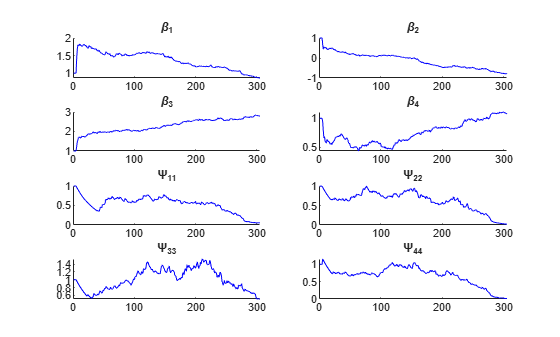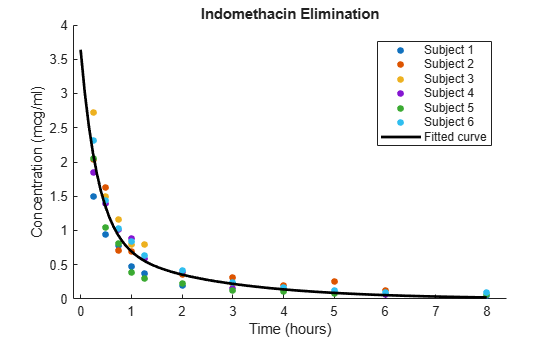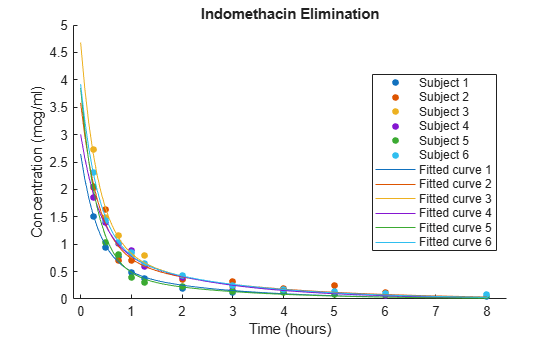# nlmefitsa

Fit nonlinear mixed-effects model with stochastic EM algorithm

## Syntax

[BETA,PSI,STATS,B] = nlmefitsa(X,Y,GROUP,V,MODELFUN,BETA0) [BETA,PSI,STATS,B] = nlmefitsa(X,Y,GROUP,V,MODELFUN,BETA0,'Name',Value) 

## Description

[BETA,PSI,STATS,B] = nlmefitsa(X,Y,GROUP,V,MODELFUN,BETA0) fits a nonlinear mixed-effects regression model and returns estimates of the fixed effects in BETA. By default, nlmefitsa fits a model where each model parameter is the sum of a corresponding fixed and random effect, and the covariance matrix of the random effects is diagonal, i.e., uncorrelated random effects.

The BETA, PSI, and other values this function returns are the result of a random (Monte Carlo) simulation designed to converge to the maximum likelihood estimates of the parameters. Because the results are random, it is advisable to examine the plot of simulation to results to be sure that the simulation has converged. It may also be helpful to run the function multiple times, using multiple starting values, or use the 'Replicates' parameter to perform multiple simulations.

[BETA,PSI,STATS,B] = nlmefitsa(X,Y,GROUP,V,MODELFUN,BETA0,'Name',Value) accepts one or more comma-separated parameter name/value pairs. Specify Name inside single quotes.

## Input Arguments

Definitions:

In the following list of arguments, the following variable definitions apply:

• n — number of observations

• h — number of predictor variables

• m — number of groups

• g — number of group-specific predictor variables

• p — number of parameters

• f — number of fixed effects

 X An n-by-h matrix of n observations on h predictor variables. Y An n-by-1 vector of responses. GROUP A grouping variable indicating to which of m groups each observation belongs. GROUP can be a categorical variable, a numeric vector, a character matrix with rows for group names, a string array, or a cell array of character vectors. V An m-by-g matrix of g group-specific predictor variables for each of the m groups in the data. These are predictor values that take on the same value for all observations in a group. Rows of V are ordered according to GRP2IDX(GROUP). Use an m-by-g cell array for V if any of the group-specific predictor values vary in size across groups. Specify [] for V if there are no group predictors. MODELFUN A handle to a function that accepts predictor values and model parameters, and returns fitted values. MODELFUN has the form YFIT = MODELFUN(PHI,XFUN,VFUN) with input arguments PHI — A 1-by-p vector of model parameters. XFUN — An l-by-h array of predictor variables where l is 1 if XFUN is a single row of Xl is ni if XFUN contains the rows of X for a single group of size nil is n if XFUN contains all rows of X. VFUN — Either A 1-by-g vector of group-specific predictors for a single group, corresponding to a single row of VAn n-by-g matrix, where the k-th row of VFUN is V(i,:) if the k-th observation is in group i. If V is empty, nlmefitsa calls MODELFUN with only two inputs. MODELFUN returns an l-by-1 vector of fitted values YFIT. When either PHI or VFUN contains a single row, that one row corresponds to all rows in the other two input arguments. For improved performance, use the 'Vectorization' parameter name/value pair (described below) if MODELFUN can compute YFIT for more than one vector of model parameters in one call. BETA0 An f-by-1 vector with initial estimates for the f fixed effects. By default, f is equal to the number of model parameters p. BETA0 can also be an f-by-REPS matrix, and the estimation is repeated REPS times using each column of BETA0 as a set of starting values.

### Name-Value Arguments

By default, nlmefitsa fits a model where each model parameter is the sum of a corresponding fixed and random effect. Use the following parameter name/value pairs to fit a model with a different number of or dependence on fixed or random effects. Use at most one parameter name with an 'FE' prefix and one parameter name with an 'RE' prefix. Note that some choices change the way nlmefitsa calls MODELFUN, as described further below.

 FEParamsSelect A vector specifying which elements of the model parameter vector PHI include a fixed effect, as a numeric vector with elements in 1:p, or as a 1-by-p logical vector. The model will include f fixed effects, where f is the specified number of elements. FEConstDesign A p-by-f design matrix ADESIGN, where ADESIGN*BETA are the fixed components of the p elements of PHI. FEGroupDesign A p-by-f-by-m array specifying a different p-by-f fixed effects design matrix for each of the m groups. REParamsSelect A vector specifying which elements of the model parameter vector PHI include a random effect, as a numeric vector with elements in 1:p, or as a 1-by-p logical vector. The model will include r random effects, where r is the specified number of elements. REConstDesign A p-by-r design matrix BDESIGN, where BDESIGN*B are the random components of the p elements of PHI. This matrix must consist of 0s and 1s, with at most one 1 per row.

The default model is equivalent to setting both FEConstDesign and REConstDesign to eye(p), or to setting both FEParamsSelect and REParamsSelect to 1:p.

Additional optional parameter name/value pairs control the iterative algorithm used to maximize the likelihood:

 CovPattern Specifies an r-by-r logical or numeric matrix PAT that defines the pattern of the random effects covariance matrix PSI. nlmefitsa computes estimates for the variances along the diagonal of PSI as well as covariances that correspond to non-zeroes in the off-diagonal of PAT. nlmefitsa constrains the remaining covariances, i.e., those corresponding to off-diagonal zeroes in PAT, to be zero. PAT must be a row-column permutation of a block diagonal matrix, and nlmefitsa adds non-zero elements to PAT as needed to produce such a pattern. The default value of PAT is eye(r), corresponding to uncorrelated random effects. Alternatively, specify PAT as a 1-by-r vector containing values in 1:r. In this case, elements of PAT with equal values define groups of random effects, nlmefitsa estimates covariances only within groups, and constrains covariances across groups to be zero. Cov0 Initial value for the covariance matrix PSI. Must be an r-by-r positive definite matrix. If empty, the default value depends on the values of BETA0. ComputeStdErrors true to compute standard errors for the coefficient estimates and store them in the output STATS structure, or false (default) to omit this computation. ErrorModel A character vector or string scalar specifying the form of the error term. Default is 'constant'. Each model defines the error using a standard normal (Gaussian) variable e, the function value f, and one or two parameters a and b. Choices are 'constant' — y = f + a*e'proportional' — y = f + b*f*e'combined' — y = f + (a+b*f)*e'exponential' — y = f*exp(a*e), or equivalently log(y) = log(f) + a*e If this parameter is given, the output STATS.errorparam field has the value a for 'constant' and 'exponential' b for 'proportional' [a b] for 'combined' ErrorParameters A scalar or two-element vector specifying starting values for parameters of the error model. This specifies the a, b, or [a b] values depending on the ErrorModel parameter. LogLikMethod Specifies the method for approximating the loglikelihood. Choices are: 'is' — Importance sampling 'gq' — Gaussian quadrature'lin' — Linearization 'none' — Omit the loglikelihood approximation (default) NBurnIn Number of initial burn-in iterations during which the parameter estimates are not recomputed. Default is 5. NChains Number c of "chains" simulated. Default is 1. Setting c>1 causes c simulated coefficient vectors to be computed for each group during each iteration. Default depends on the data, and is chosen to provide about 100 groups across all chains. NIterations Number of iterations. This can be a scalar or a three-element vector. Controls how many iterations are performed for each of three phases of the algorithm: simulated annealingfull step size reduced step size Default is [150 150 100]. A scalar is distributed across the three phases in the same proportions as the default. NMCMCIterations Number of Markov Chain Monte Carlo (MCMC) iterations. This can be a scalar or a three-element vector. Controls how many of three different types of MCMC updates are performed during each phase of the main iteration: full multivariate updatesingle coordinate updatemultiple coordinate update Default is [2 2 2]. A scalar value is treated as a three-element vector with all elements equal to the scalar. OptimFun Optimization function for the estimation process that maximizes a likelihood function, specified as 'fminsearch' (default) or 'fminunc'. Use of 'fminunc' requires Optimization Toolbox™. The fminsearch function uses a direct search method that uses only function evaluations. The fminunc (Optimization Toolbox) function uses gradient methods and is generally more efficient for an optimization problem that maximizes the likelihood function. Options A structure created by a call to statset. nlmefitsa uses the following statset parameters: 'DerivStep' — Relative difference used in finite difference gradient calculation. May be a scalar, or a vector whose length is the number of model parameters p. The default is eps^(1/3).Display — Level of display during estimation. 'off' (default) — Displays no information'final' — Displays information after the final iteration of the estimation algorithm'iter' — Displays information at each iteration FunValCheck 'on' (default) — Check for invalid values (such as NaN or Inf) from MODELFUN'off' — Skip this check OutputFcn — Function handle specified using @, a cell array with function handles or an empty array. nlmefitsa calls all output functions after each iteration. See nlmefitoutputfcn.m (the default output function for nlmefitsa) for an example of an output function. ParamTransform A vector of p-values specifying a transformation function f() for each of the p parameters: XB = ADESIGN*BETA + BDESIGN*B PHI = f(XB) Each element of the vector must be one of the following integer codes specifying the transformation for the corresponding value of PHI: 0: PHI = XB (default for all parameters) 1: log(PHI) = XB2: probit(PHI) = XB3: logit(PHI) = XB Replicates Number REPS of estimations to perform starting from the starting values in the vector BETA0. If BETA0 is a matrix, REPS must match the number of columns in BETA0. Default is the number of columns in BETA0. Vectorization Determines the possible sizes of the PHI, XFUN, and VFUN input arguments to MODELFUN. Possible values are: 'SinglePhi' — MODELFUN is a function (such as an ODE solver) that can only compute YFIT for a single set of model parameters at a time, i.e., PHI must be a single row vector in each call. nlmefitsa calls MODELFUN in a loop if necessary using a single PHI vector and with XFUN containing rows for a single observation or group at a time. VFUN may be a single row that applies to all rows of XFUN, or a matrix with rows corresponding to rows in XFUN.'SingleGroup' — MODELFUN can only accept inputs corresponding to a single group in the data, i.e., XFUN must contain rows of X from a single group in each call. Depending on the model, PHI is a single row that applies to the entire group, or a matrix with one row for each observation. VFUN is a single row. 'Full' — MODELFUN can accept inputs for multiple parameter vectors and multiple groups in the data. Either PHI or VFUN may be a single row that applies to all rows of XFUN, or a matrix with rows corresponding to rows in XFUN. Using this option can improve performance by reducing the number of calls to MODELFUN, but may require MODELFUN to perform singleton expansion on PHI or V. The default for 'Vectorization' is 'SinglePhi'. In all cases, if V is empty, nlmefitsa calls MODELFUN with only two inputs.

## Output Arguments

 BETA Estimates of the fixed effects PSI An r-by-r estimated covariance matrix for the random effects. By default, r is equal to the number of model parameters p. STATS A structure with the following fields: logl — The maximized loglikelihood for the fitted model; empty if the LogLikMethod parameter has its default value of 'none' rmse — The square root of the estimated error variance (computed on the log scale for the exponential error model)errorparam — The estimated parameters of the error variance model aic — The Akaike information criterion (empty if logl is empty), calculated as aic = –2 * logl + 2 * numParam, where logl is the maximized loglikelihood.numParam is the number of fitting parameters, including the degree of freedom for covariance matrix of the random effects, the number of fixed effects and the number of parameters of the error model. bic — The Bayesian information criterion (empty if logl is empty), calculated as bic = -2*logl + log(M) * numParam M is the number of groups.logl and numParam are defined as in aic. Note that some literature suggests that the computation of bic should be , bic = -2*logl + log(N) * numParam, where N is the number of observations. To adjust the value of the output you can redefine bic as follows: bic = bic - numel(unique(group)) + numel(Y)sebeta — The standard errors for BETA (empty if the ComputeStdErrors parameter has its default value of false)covb — The estimated covariance of the parameter estimates (empty if ComputeStdErrors is false)dfe — The error degrees of freedompres — The population residuals (y-y_population), where y_population is the population predicted valuesires — The population residuals (y-y_population), where y_population is the individual predicted valuespwres — The population weighted residualscwres — The conditional weighted residualsiwres — The individual weighted residuals

## Examples

collapse all

load indomethacin 

Fit a model to data on concentrations of the drug indomethacin in the bloodstream of six subjects over eight hours.

model = @(phi,t)(phi(:,1).*exp(-phi(:,2).*t)+phi(:,3).*exp(-phi(:,4).*t)); phi0 = [1 1 1 1]; xform = [0 1 0 1]; % log transform for 2nd and 4th parameters [beta,PSI,stats,br] = nlmefitsa(time,concentration,... subject,[],model,phi0,'ParamTransform',xform) 
beta = 0.8563 -0.7950 2.7744 1.0772 PSI = 0.0529 0 0 0 0 0.0220 0 0 0 0 0.4762 0 0 0 0 0.0120 stats = struct with fields: logl: [] aic: [] bic: [] sebeta: [] dfe: 57 covb: [] errorparam: 0.0809 rmse: 0.0775 ires: [66x1 double] pres: [66x1 double] iwres: [66x1 double] pwres: [66x1 double] cwres: [66x1 double] br = -0.2255 0.0063 0.1600 0.1773 -0.3269 0.1157 0.0350 -0.1384 0.0058 0.0431 0.0093 -0.0453 -0.7557 -0.0550 0.8736 -0.7875 0.5304 0.1727 -0.0010 -0.0198 0.0137 -0.0757 0.0478 -0.0076Plot the data along with an overall population fit

clf phi = [beta(1), exp(beta(2)), beta(3), exp(beta(4))]; h = gscatter(time,concentration,subject); xlabel('Time (hours)') ylabel('Concentration (mcg/ml)') title('{\bf Indomethacin Elimination}') xx = linspace(0,8); line(xx,model(phi,xx),'linewidth',2,'color','k')Plot individual curves based on random-effect estimates.

for j=1:6 phir = [beta(1)+br(1,j), exp(beta(2)+br(2,j)), ... beta(3)+br(3,j), exp(beta(4)+br(4,j))]; line(xx,model(phir,xx),'color',get(h(j),'color')) end## Algorithms

In order to estimate the parameters of a nonlinear mixed effects model, we would like to choose the parameter values that maximize a likelihood function. These values are called the maximum likelihood estimates. The likelihood function can be written in the form

$p\left(y|\beta ,{\sigma }^{2},\Sigma \right)=\int p\left(y|\beta ,b,{\sigma }^{2}\right)p\left(b|\Sigma \right)db$

where

• y is the response data

• β is the vector of population coefficients

• σ2 is the residual variance

• ∑ is the covariance matrix for the random effects

• b is the set of unobserved random effects

Each p() function on the right-hand-side is a normal (Gaussian) likelihood function that may depend on covariates.

Since the integral does not have a closed form, it is difficult to find parameters that maximize it. Delyon, Lavielle, and Moulines  proposed to find the maximum likelihood estimates using an Expectation-Maximization (EM) algorithm in which the E step is replaced by a stochastic procedure. They called their algorithm SAEM, for Stochastic Approximation EM. They demonstrated that this algorithm has desirable theoretical properties, including convergence under practical conditions and convergence to a local maximum of the likelihood function. Their proposal involves three steps:

1. Simulation: Generate simulated values of the random effects b from the posterior density p(b|Σ) given the current parameter estimates.

2. Stochastic approximation: Update the expected value of the loglikelihood function by taking its value from the previous step, and moving part way toward the average value of the loglikelihood calculated from the simulated random effects.

3. Maximization step: Choose new parameter estimates to maximize the loglikelihood function given the simulated values of the random effects.

## References

 Delyon, B., M. Lavielle, and E. Moulines, "Convergence of a stochastic approximation version of the EM algorithm." Annals of Statistics, 27, 94-128, 1999.

 Mentré, F., and M. Lavielle, "Stochastic EM Algorithms in Population PKPD analyses." American Conference on Pharmacometrics, 2008.